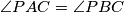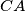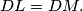### IMO Shortlist 1982 problem 9

Kvaliteta:
Avg: 0.0
Težina:
Avg: 0.0
Let$ABC$ be a triangle, and let$P$ be a point inside it such that$\angle PAC = \angle PBC$. The perpendiculars from$P$ to$BC$ and$CA$ meet these lines at$L$ and$M$, respectively, and$D$ is the midpoint of$AB$. Prove that$DL = DM.$
Source: Međunarodna matematička olimpijada, shortlist 1982